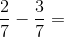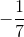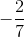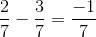# SSAT Upper Level Math : How to subtract fractions

## Example Questions

### Example Question #1 : How To Subtract Fractions

Janice had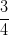of a cup of milk. If she used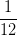cup to make some scrambled eggs, how much of a cup of milk does she have left?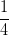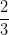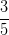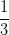Explanation:

Subtract the two fractions. To subtract them, they must have a common denominator. Multiply the numerator and denominator ofbyto create a common denominator of, then subtract the numerators and reduce the resulting fraction.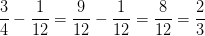### Example Question #2 : How To Subtract Fractions

What is the result of this operation?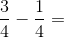Explanation:

Since the denominators are exactly the same, we can just subtract the tops.

So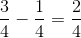By reducing we get### Example Question #3 : How To Subtract Fractions

What is the result of this operation?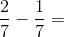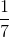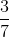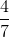Explanation:

Since the denominators are the same, we can just subtract the numerators.

So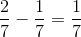### Example Question #4 : How To Subtract Fractions

What is the result of this operation?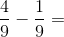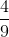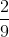Explanation:

Since the denominators are the same, we can just subtract the numerators.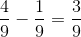By reducing our result, we get### Example Question #5 : How To Subtract Fractions

What is the result of this operation?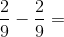Explanation:

Since the denominators are the same, we can just subtract the numerators.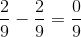The result can just be reduced to### Example Question #6 : How To Subtract Fractions

What is the result of the operation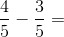Explanation:

Since the denominators are the exact same, we can just subtract the numerators.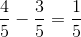### Example Question #7 : How To Subtract Fractions

What is the result of this operation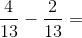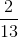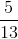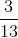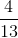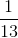Explanation:

Since the denominators are the exact same, we can just subtract the numerators.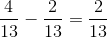### Example Question #8 : How To Subtract Fractions

What is the result of this operation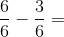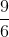Explanation:

Since the denominators are the same, we can just subtract the numerators.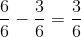which we can reduce to### Example Question #9 : How To Subtract Fractions

What is the result of this operation?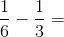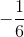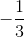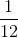Explanation:

Since the denominators are different, we need to transform them into the same denominator.

Sobecomes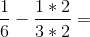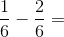Now subtract the numerators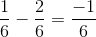### Example Question #10 : How To Subtract Fractions

What is the result of this operation